# Precalculus : Logarithmic Functions

## Example Questions

1 3 Next →

### Example Question #1 : Graph Logarithms

Evaluate the following logarithm: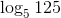Possible Answers:Correct answer:Explanation:

The notation of this logarithm is asking "5 to what power equals 125?" If we set this up mathematically, we can simplify either side until it is readily apparent what the value of x is, which is the value of the logarithm: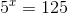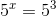So to answer our question, 5 to the power of 3 is 125, so the answer is 3.

### Example Question #11 : Graph Logarithms

Evaluate: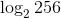Possible Answers:Correct answer:Explanation:

To evaluate, you must convert 256 to a power of 2. Then the log and 2 will cancel to leave you with your answer.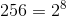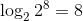### Example Question #12 : Graph Logarithms

Which of these is a correct method for evaluating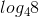?

Possible Answers: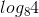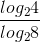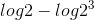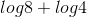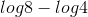Correct answer:Explanation:

When evaluating a log you are asking what power your base must be raised by to get your argument.

If you cannot directly figure that out you can use a change of base formula instead.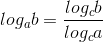### Example Question #13 : Graph Logarithms

Evaluate this logarithm: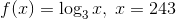Possible Answers: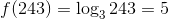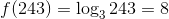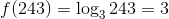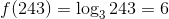Correct answer:Explanation:

It is important to remember a logarithm is really just an exponent. In fact when you see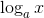remember that the expression is only asking what exponent must "a" be raised to in order to obtain "x"!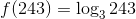Now that we have substituted the value of x reread the problem by the rule above. What power must 3 be raised to in order to obtain 243?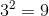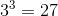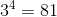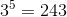5 is the power to which 3 must be raised to obtain 243.

### Example Question #14 : Graph Logarithms

Evaluate the logarithm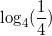Possible Answers: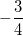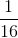Correct answer:Explanation:

Given the property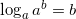and because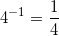we obtain that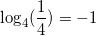### Example Question #1211 : Pre Calculus

Evaluate to four decimal places: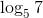Possible Answers: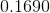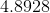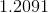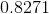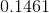Correct answer:Explanation:

Using a calculator, evaluate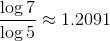### Example Question #16 : Graph Logarithms

Evaluate to 4 decimal places: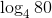Possible Answers: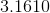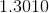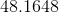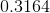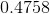Correct answer:Explanation:

To evaluate, use a calculator to find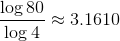### Example Question #17 : Graph Logarithms

Evaluate the following: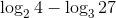Possible Answers:Correct answer:Explanation:

To solve, simply find the matching base and use properties of logs to simplify.

Some properties of logs include the following:

1)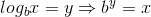2)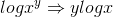3)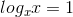Thus,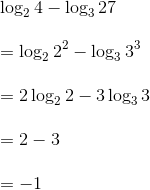### Example Question #11 : Graph Logarithms

Evaluate the following: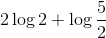Possible Answers: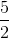Correct answer:Explanation:

To solve, remember the following rules for logarithms.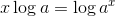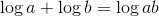Thus,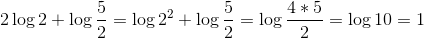Remember, if a base isn't specified, it is 10.

1 3 Next →

### All Precalculus Resources